# Calculating Voltage Amplitude of the output of an integrator op-amp

#### ProfessorSciFi

Joined Oct 31, 2019
6
Hello,

I am trying to calculate the voltage output amplitude of the integrator. I understand that integrating a square-wave creates a triangle, and integrating a triangle creates a sine-wave, and a sinewave creates a cosine-wave. I understand corner frequency is calculated using 1/(2pi(R2)(C)). But I am having a really hard time figuring out the amplitude of the output.

The attached plot shows the input and output waveforms at approximately the -3dB point with is approximately 1kHz.

Any direction is greatly appreciated,
Daniel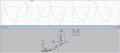#### Papabravo

Joined Feb 24, 2006
20,378
Hello,

I am trying to calculate the voltage output amplitude of the integrator. I understand that integrating a square-wave creates a triangle, and integrating a triangle creates a sine-wave, and a sinewave creates a cosine-wave. I understand corner frequency is calculated using 1/(2pi(R2)(C)). But I am having a really hard time figuring out the amplitude of the output.

The attached plot shows the input and output waveforms at approximately the -3dB point with is approximately 1kHz.

Any direction is greatly appreciated,
Daniel
You wrote:
"integrating a triangle creates a sine-wave..."
This is not factually correct. Integrating a a linear polynomial produces a quadratic polynomial. If it produced a sine-wave then differentiating a sine wave would produce a linear function and that is manifestly WRONG!

ETA: The circuit you have is not strictly an integrator. It is known as a lossy integrator because at low frequency the capacitor resembles an open circuit, and the gain is given by the feedback resistor over the input resistor.

See the following: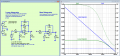It looks like the two are the same for frequencies of 2+ kHz and above.

Last edited:

#### dcbingaman

Joined Jun 30, 2021
949
Technically this is an integrator with negative gain. Not sure if that matters for you. The transfer function I get for it in the frequency domain is just: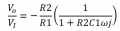Where Vo is the output voltage and Vi is the input voltage

You did not specify what input waveform you are integrating. Because the answer to what the peak to peak output voltage will be depends a lot on the type of waveform at the input.

Assuming it is a simple sine wave you can simply apply the above formula. Just be careful to realize you have a complex number in the denominator, so the answer will be a complex result.

For low frequencies where omega is much smaller than R2C1 the right hand factor goes to 1 and the output is just a constant of -R2/R1. For really high frequencies where omega is much greater than R2C1 the output is just:

j/(R1C1w)

Notice this is an imaginary number as j is in the numerator and you thus get a 90 degree phase shift as expected.

If you are dealing with a square wave, triangle wave or some other 'arbitrary' input waveform the output is much more difficult to predict.

Last edited:

#### DickCappels

Joined Aug 21, 2008
10,067
About 100 years ago a pulse was used to generate a sawtooth in an integrator and the it was integrated again to make a parabola. The rates and therefore the amplitudes of the waveforms were adjustable electrically and signals applied to deflection and video circuits of video cameras and monitors to correct geometric distortion and variations in brightness of the image.

That only lasted until solid state image sensors came into wide use.

#### neonstrobe

Joined May 15, 2009
189
I think the OP was planning to integrate a square wave - and again to get a sinewave. But integrating a triangle wave cannot yield a sinewave as there is an abrupt transition at each apex. Filtering (perhaps this might be considered a series of integrators) could potentially yield a sinewave but that would need a filter design not just a couple of integrators.
If the input were a square wave then it is fairly easy to predict the output of a simple integrator (assuming that the frquency is higher than the lossy integrator low-frequency divergence point). Just use dV/dt=i/C where i is the input current set by Vin/Rin and C the integrating capacitor. You know t from the frequency but be careful as there is a factor of 2 here to consider as the period changes - that's a task I'll leave for the OP to work out.
If you then want to integrate the triangle wave you'll get a second order calculation which won't be a sinewave because the i in the basic equation becomes time-dependent.
If the aim is to generate a sinewave from the square wave look up filter design.

#### LvW

Joined Jun 13, 2013
1,667
Any direction is greatly appreciated,
Daniel
Perhaps it is not clear to you that the circuit shown is NOT an integrator.
It rather is an inverting 1st-order lowpass which can be used as an integrating device within a pretty small frequency range only (far above the 3dB-cutoff frequency).
In fact, the phase shift of 90 deg (required for an integrator) does exist at one single frequency only.
This does even apply for the so-called "ideal" integrator without any resistor in the feedback loop. However, such an integrator (without any stabilizing dc negative feedback) must not be used as a stand-alone unit - but only as one block within an overall feedback loop (filters, oscillators,..)

#### DickCappels

Joined Aug 21, 2008
10,067
@LvW has a point, you don't need an integrator, just a good low pass filter.

A good way to get something that looks a lot like a sine wave is to make a square wave the follow it with a lot of filtering to reduce the harmonic content. Back in ancient times (before op amps) I would use a two transistor multivibrator to make square waves then follow it with a three pole RC filter at much less than the multivibrator's frequency. Worked fine for my purpose, which was exploring adding individually modulated sidebands to an AM transmitter.

Today I might use one RC to knock off the really high frequency harmonics, followed by a two pole active filter.

#### Papabravo

Joined Feb 24, 2006
20,378
Here is an example: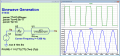You may not have realized this in your simulation, but when you do not specify rise and fall times for a PULSE source, LTspice inserts default values. This is to avoid signals with infinite derivatives (aka vertical slopes). In this simulation I am explicitly setting the Transition Time (TT) to the approximate default value for this on time and period. I have also adjusted the ON-time so that the transitions are allocated equally to the on time and the off time. With a 3rd order filter, the output looks pretty good, and this is confirmed by the FFT.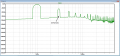•DickCappels

#### LvW

Joined Jun 13, 2013
1,667
Here is a quite simple method for triangle-sine conversion which works for a broad range of frequencies (constant amplitudes):
Use the nonlinear (hyperbel-tan) transfer characteristics of the known two-transistor differential amplifier.
Example: For a triangle amplitude of app. 80mV he THD of the sinewave will be app 1.3% only.